Where definitions are stored

The order that definitions are stored and changing the order of the  definitions is discussed in sections 2.4.7 and 2.4.13 of The Mathematica Book.

In some cases you might also need to write a function that will  automatically examine the definitions of a certain symbol.  Your function  might then return part of those definitions or automatically make changes to  some of the definitions. In order to do this you need to know where the  definitions are stored.  Mathematica stores definitions as DownValues, UpValues, OwnValues, NValues, SubValues, FormatValues, DefaultValues, and  Messages.  Except for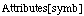and Options[symb] anything that affects evaluation of expressions  involving symb are stored in one of these places and they are each discussed  below.

If you evaluate (??symb) all information asssociated with symb is  displayed, except the only message displayed is (symb::usage) and only if  symb has a usage message.

DownValues

Definitions made using (f[args] := rhs) are stored in DownValues[f] as
demonstrated with the next cell.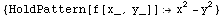Definitions made using (f[args] = rhs) are also in DownValues[f] as
demonstrated with the next cell.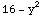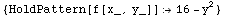UpValues

Definitions made using (f/: lhs ^:= rhs) are stored in UpValues[f] as
demonstrated with the next cell.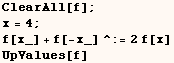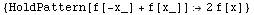Definitions made using (f/: lhs := rhs) are also stored in UpValues[f].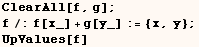On rare occasions it's wise to store definitions using (f[args] ^= rhs) and
these definitions are also stored in UpValues[f].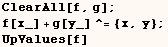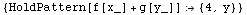Use of (f/: lhs = rhs) isn't even documented, but it seems to give the
expected result and it also makes a definition stored in  UpValues[f].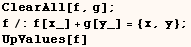OwnValues

Definitions for (symb=expr) and (symb:=expr) are stored in OwnValues[symb].
The next cell assigns a value to expr.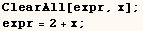Next we see the above definition is stored in OwnValues[expr].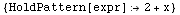NValues

Definitions for N[f[args]] are stored in NValues[f].  The next cell makes a
definition for  N[f[x_,y_]].The above definition isn't used to evaluate the next cell because N wasn't
used.In the next cell our definition for N[f[x_,y_]] is used.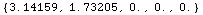Our definition for N[f[x_,y_]] is stored in NValues[f].SubValues

Definitions for  f[arg1][args]  are stored in  SubValues[f].  This is
demonstrated with the next cell.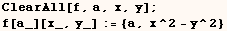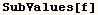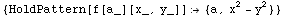The next cell has the effect of defining a function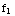and the definition is stored in SubValues[Subscript].
The definition is  stored in SubValues[Subscript] because the head of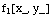is  Subscript[f,1].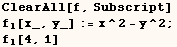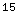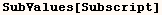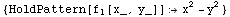FormatValues

A user once wrote to the MathGroup asking how one could ensure rational  numbers greater than one are displayed as improper fractions.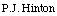of Wolfram Research gave the solution below to change the way rational  numbers are formated.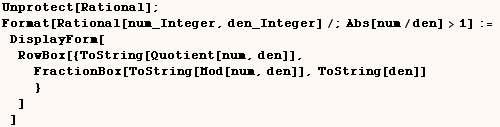After evaluating the cell above the output of the next cell is formatted as
an improper fraction.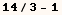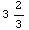Below we see that the above definition is stored in  FormatValues[Rational].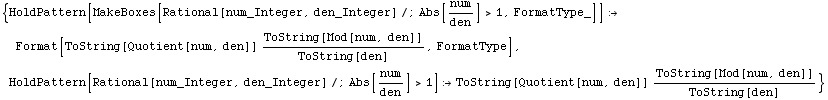Before you continue you might want to evaluate the next cell to restore the
default formatting of rational numbers.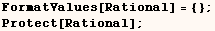MakeBoxes is similar to Format and FormatValues, and definitions using  MakeBoxes are stored in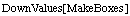or possibly in UpValues for the type of expression being formatted.

DefaultValues

Anything stored using Default[f], Default[f,i], or Default[f,i,n] is  stored in DefaultValues[f].  The built-in functions Plus, Times and Power  have defaults stored as DefaultValues as the next cell shows. Use of Default was explained in an earlier section.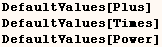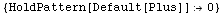Options are also stored in DefaultValues as demonstrated with the next cell.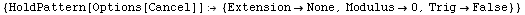You could use DefaultValues to see what options a function has or change  the options, but I can't see why anyone would want to do this.  Since options  are stored as DefaultValues you should be cautious about usingto delete all defaults.

In the next cell I assign a value to Default[f1,2] and I give f1  options.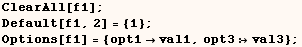The next cell shows that the previous definitions are stored in
DefaultValues[f1].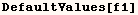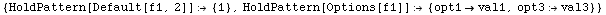Messages

The next cell makes Messages related to the symbol foo.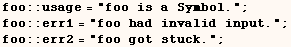At times you might want to check on or manipulate the messages above.  The
next cell gives us the list of messages as they are stored.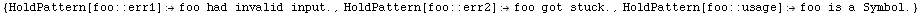Below we see that evaluating (??foo) displays the usage message for foo, but
not the error messages for foo.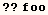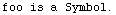Created by Mathematica  (May 17, 2004)